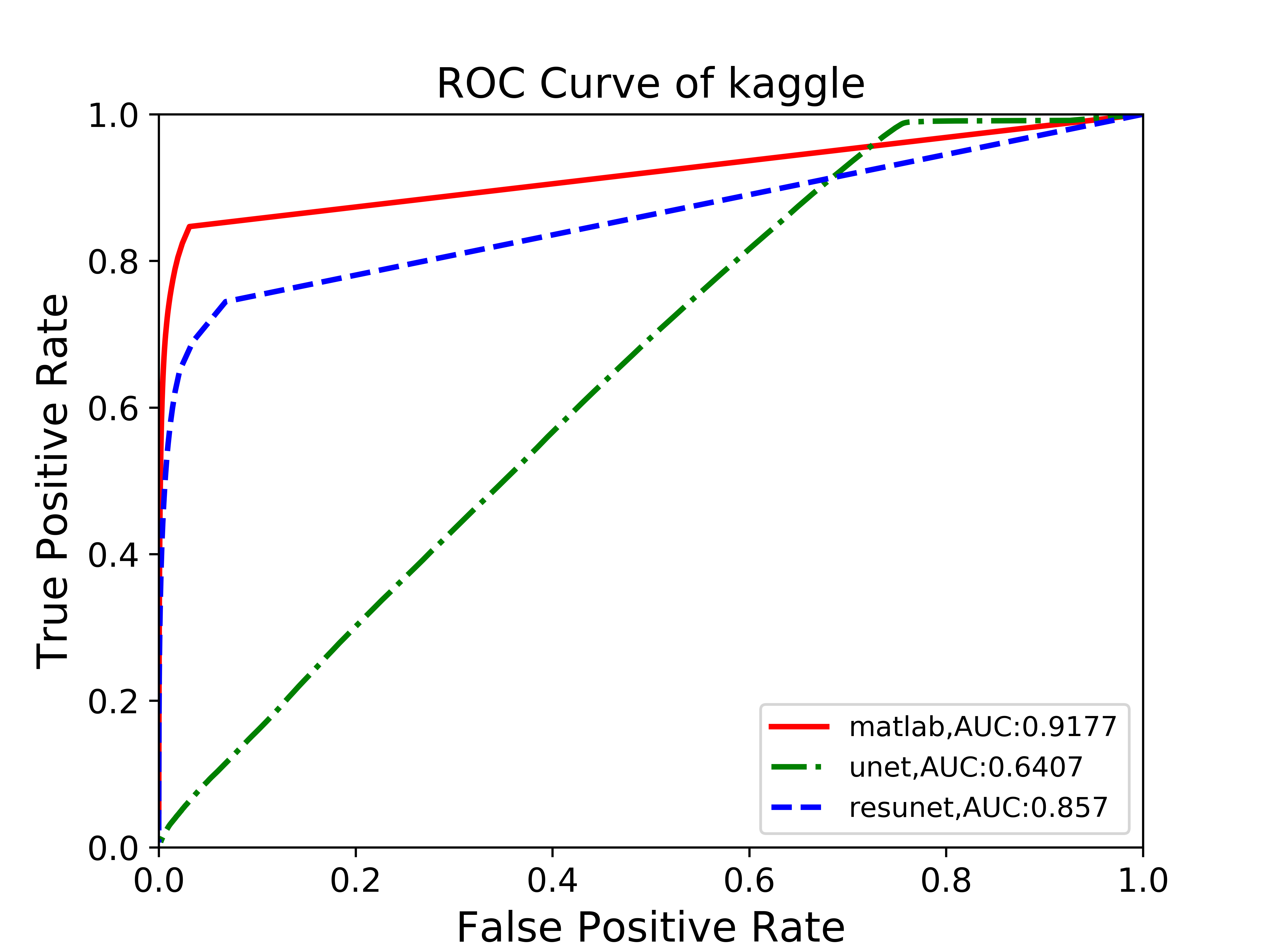# ChengruZhu / AutoMetric

draw ROC,PR curve and calculate AUC MAP IoU for image semantic segmentation problem

Geek Repo

Github PK Tool

# autometric

`autometric` is to help draw ROC,PR curves for image semantic segmentation problem.When you have groundtruth image and predict probabilistic image,this package can draw ROC,PR curves and save them to your savepath.It can also calculate FPR (False Positive Rate) TPR(True Positive Rate) AUC(Area under Curve) MAP(Mean Average Precision) IoU(Intersection over Union) at the same time.

## Install

Now you can use pip to install autometric,try `pip install autometric`

requirements:

• python 3.0+
• opencv_python
• matplotlib
• numpy

## description

`autometric` include the following function/class:

• `CollectData`: a `class` to calculate FPR,TPR,AUC,MAP,IOU
• `DrawCurve`: a `class` to draw and save ROC PR curves
• `fileList`: a `function` to collect file to a list
• `drawCurve`: a `function` to draw curves and calculate parameters.Read example.py to get an idea of how to use!

if you right use the package,you can see the roc curve like below: